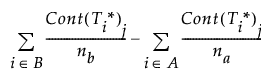Publication date: 11/10/2021

## Score Plot Group Comparisons

For the score plot group comparisons, the relative score contribution for variable j is the difference between the average contribution in group B and the average contribution in group A:where

T*i = the ith row of the score matrix with columns corresponding to the dimensions shown in the score plot.

A = the set of observations in group A

B = the set of observations in group B

na = the number of observations in group A

nb = the number of observations in group B.